Examples

Chapter 7 Class 11 Binomial Theorem
Serial order wise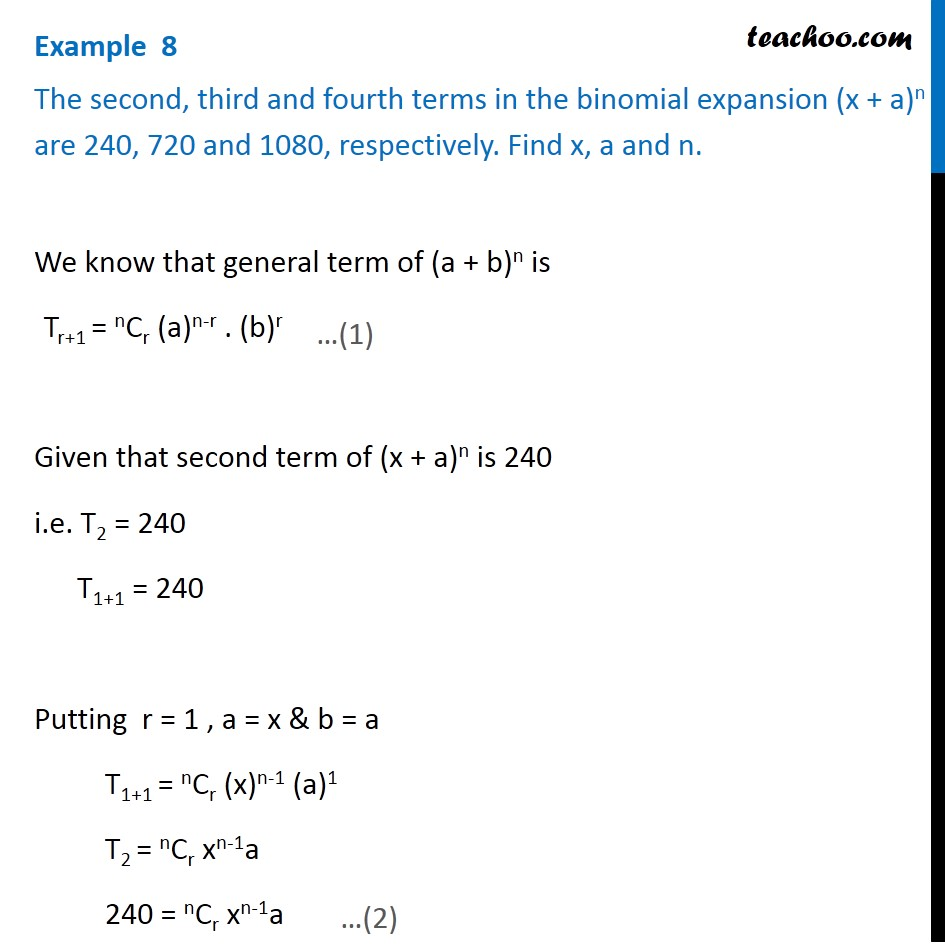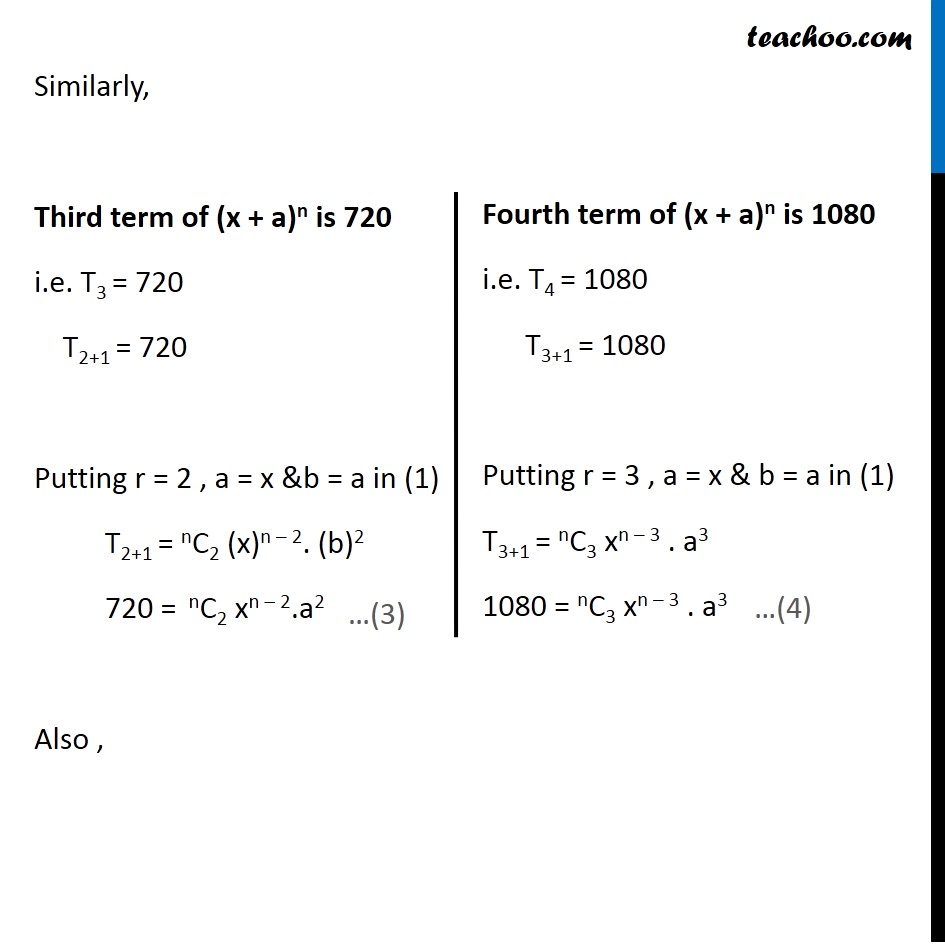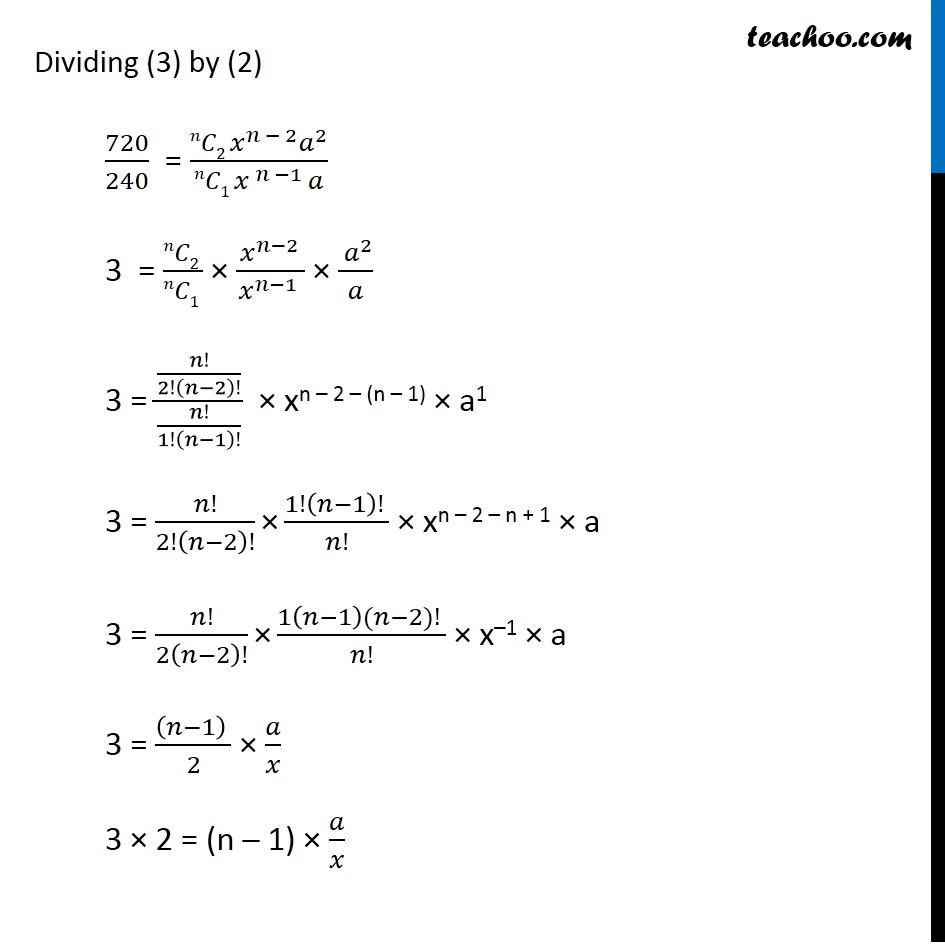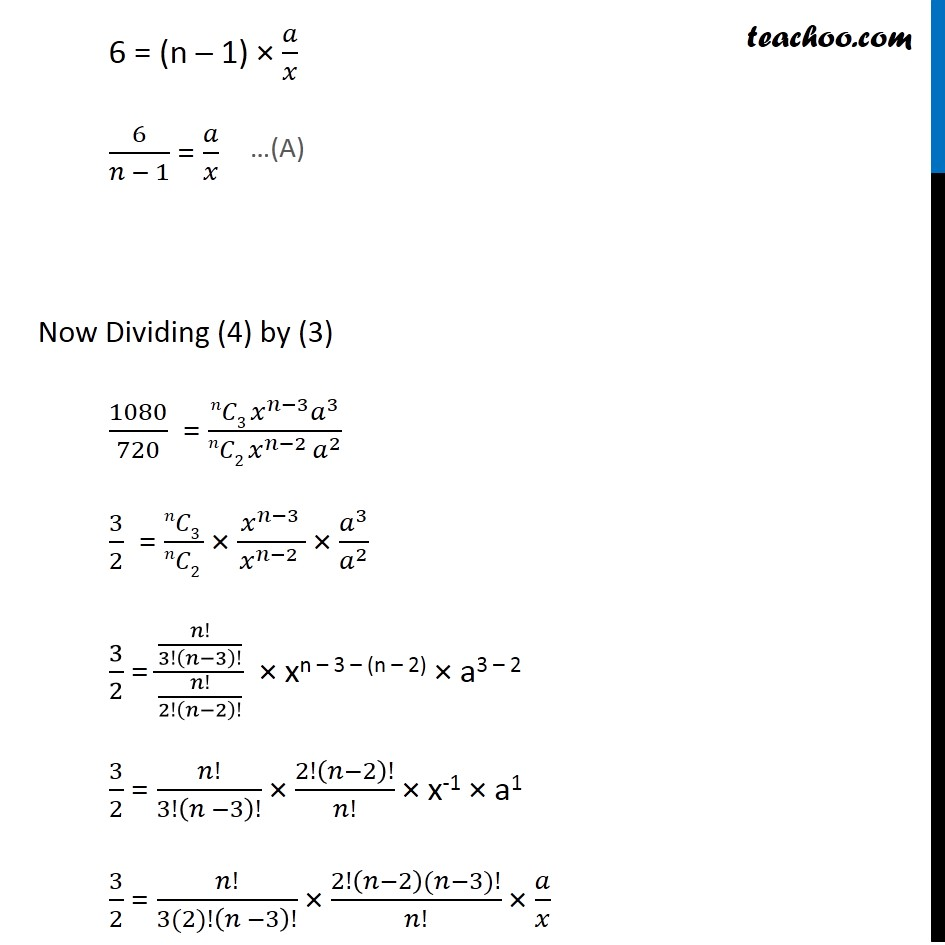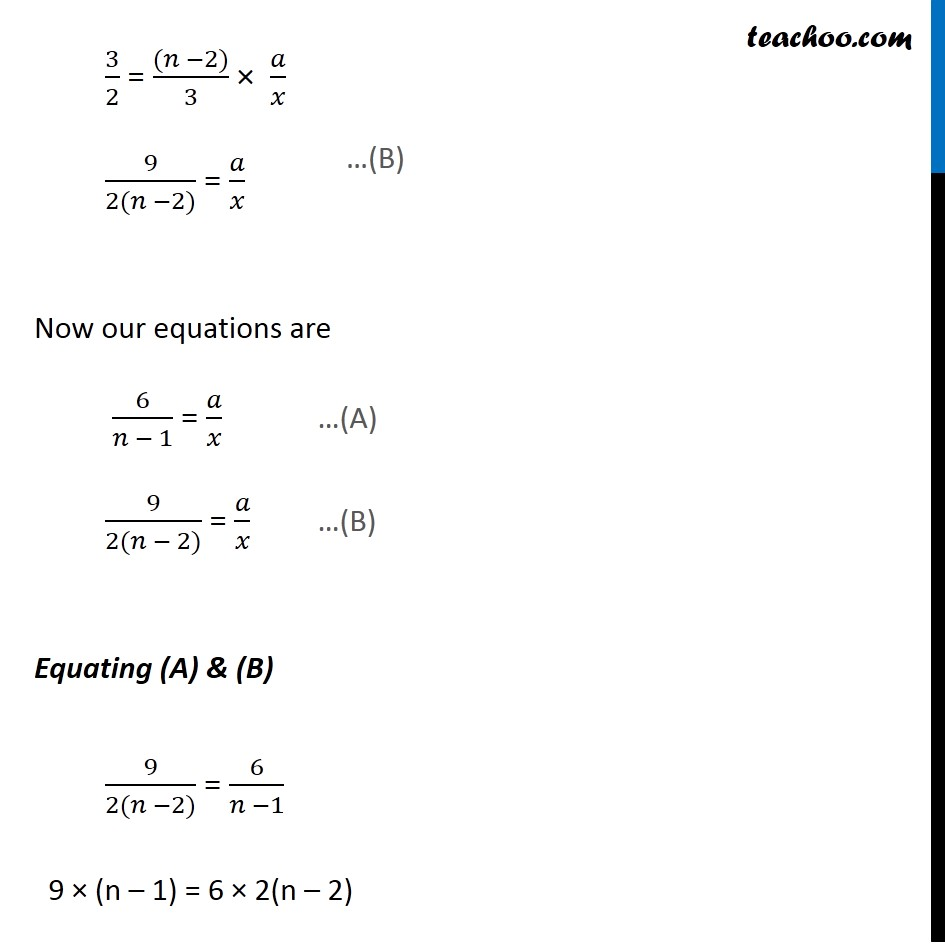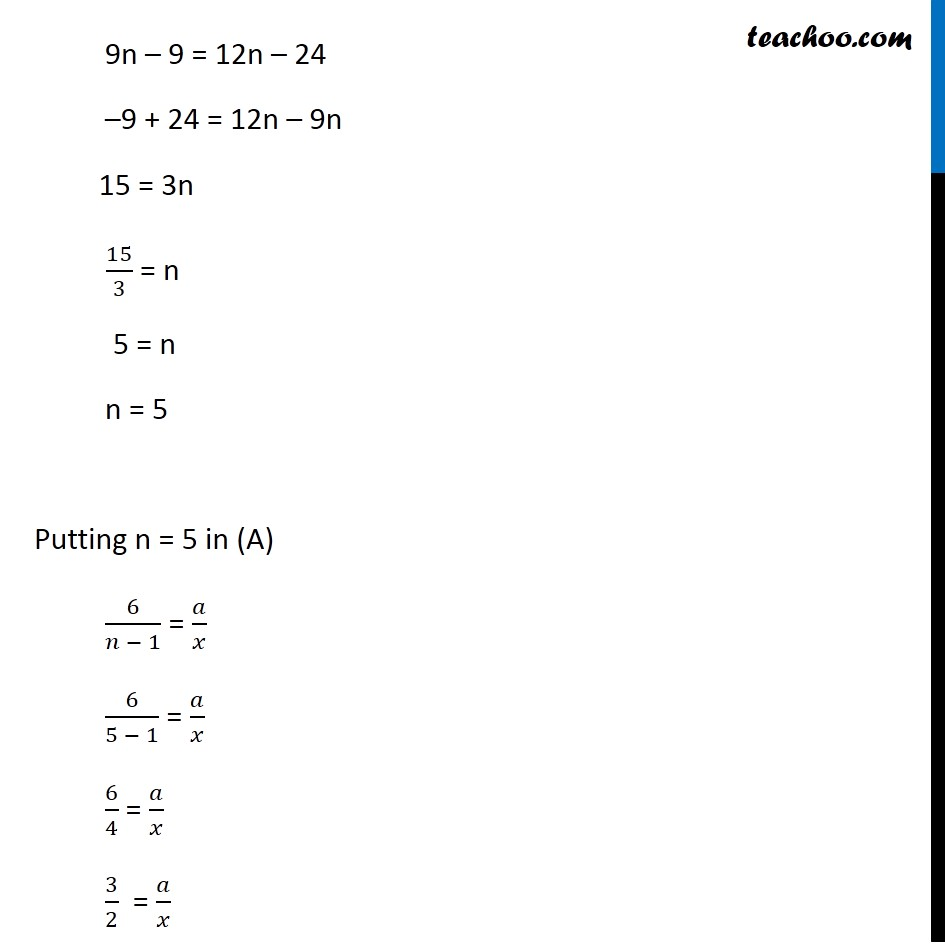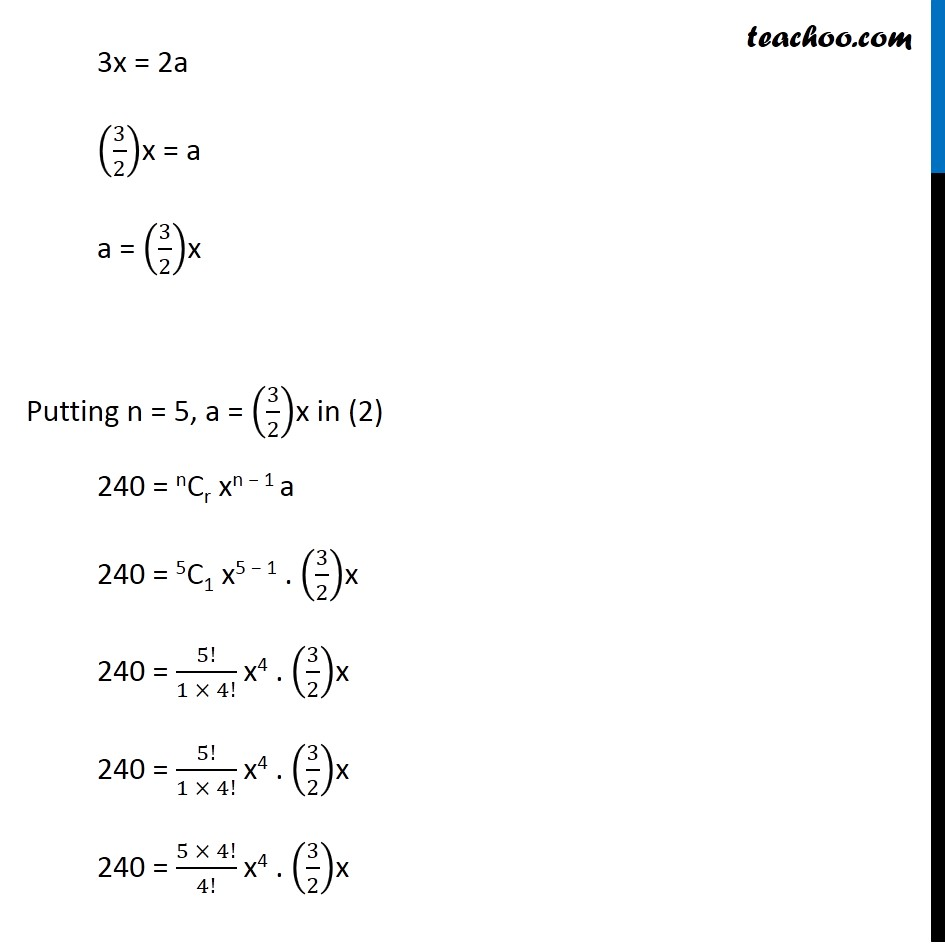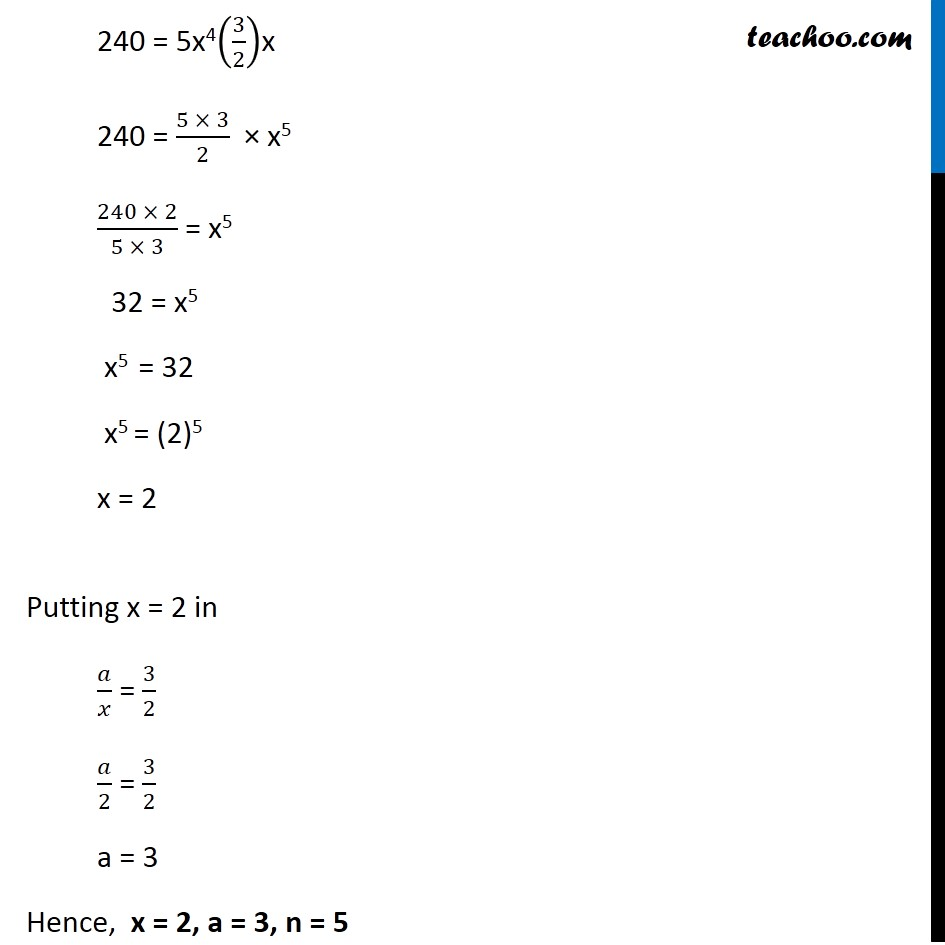Learn in your speed, with individual attention - Teachoo Maths 1-on-1 Class

### Transcript

Question 4 The second, third and fourth terms in the binomial expansion (x + a)n are 240, 720 and 1080, respectively. Find x, a and n. We know that general term of (a + b)n is Tr+1 = nCr (a)n-r . (b)r Given that second term of (x + a)n is 240 i.e. T2 = 240 T1+1 = 240 Putting r = 1 , a = x & b = a T1+1 = nCr (x)n-1 (a)1 T2 = nCr xn-1a 240 = nCr xn-1a Similarly, Third term of (x + a)n is 720 i.e. T3 = 720 T2+1 = 720 Putting r = 2 , a = x &b = a in (1) T2+1 = nC2 (x)n – 2. (b)2 720 = nC2 xn – 2.a2 Also , Fourth term of (x + a)n is 1080 i.e. T4 = 1080 T3+1 = 1080 Putting r = 3 , a = x & b = a in (1) T3+1 = nC3 xn – 3 . a3 1080 = nC3 xn – 3 . a3 Dividing (3) by (2) 720/240 = (𝑛𝐶2 𝑥^(𝑛 − 2) 𝑎^2)/(𝑛𝐶1 〖𝑥 〗^(𝑛 −1 ) 𝑎) 3 = (𝑛𝐶2 )/(𝑛𝐶1 ) × ( 𝑥^(𝑛−2) )/𝑥^(𝑛−1 ) × ( 𝑎^2)/𝑎 3 = (𝑛!/2!(𝑛−2)!)/(𝑛!/1!(𝑛−1)!) × xn – 2 – (n – 1) × a1 3 = 𝑛!/2!(𝑛−2)! "×" (1!(𝑛−1)! )/𝑛! × xn – 2 – n + 1 × a 3 = 𝑛!/2(𝑛−2)! "×" (1(𝑛−1)(𝑛−2)! )/𝑛! × x–1 × a 3 = ((𝑛−1) )/2 × 𝑎/𝑥 3 × 2 = (n – 1) × 𝑎/𝑥 6 = (n – 1) × 𝑎/𝑥 6/(𝑛 − 1) = 𝑎/𝑥 Now Dividing (4) by (3) 1080/720 = (𝑛𝐶3 𝑥^(𝑛−3) 𝑎^3)/(𝑛𝐶2 〖𝑥^(𝑛−2 ) 𝑎〗^2 ) 3/2 = (𝑛𝐶3 )/(𝑛𝐶2 ) × ( 𝑥^(𝑛−3) )/𝑥^(𝑛−2 ) × 𝑎^3/𝑎^2 3/2 = (𝑛!/3!(𝑛−3)!)/(𝑛!/2!(𝑛−2)!) × xn – 3 – (n – 2) × a3 – 2 3/2 = 𝑛!/3!(𝑛 −3)! × 2!(𝑛−2)!/𝑛! × x-1 × a1 3/2 = 𝑛!/(3(2)!(𝑛 −3)!) × (2!(𝑛−2)(𝑛−3)!)/𝑛! × 𝑎/𝑥 3/2 = ((𝑛 −2))/3 × 𝑎/𝑥 9/(2(𝑛 −2)) = 𝑎/𝑥 Now our equations are 6/(𝑛 − 1) = 𝑎/𝑥 …(A) 9/(2(𝑛 − 2)) = 𝑎/𝑥 …(B) Equating (A) & (B) 9/(2(𝑛 −2)) = 6/(𝑛 −1) 9 × (n – 1) = 6 × 2(n – 2) 9n – 9 = 12n – 24 –9 + 24 = 12n – 9n 15 = 3n 15/3 = n 5 = n n = 5 Putting n = 5 in (A) 6/(𝑛 − 1) = 𝑎/𝑥 6/(5 − 1) = 𝑎/𝑥 6/4 = 𝑎/𝑥 3/2 = 𝑎/𝑥 3x = 2a (3/2)x = a a = (3/2)x Putting n = 5, a = (3/2)x in (2) 240 = nCr xn − 1 a 240 = 5C1 x5 − 1 . (3/2)x 240 = 5!/(1 × 4!) x4 . (3/2)x 240 = 5!/(1 × 4!) x4 . (3/2)x 240 = (5 × 4!)/4! x4 . (3/2)x 240 = 5x4(3/2)x 240 = (5 × 3)/2 × x5 (240 × 2)/(5 × 3) = x5 32 = x5 x5 = 32 x5 = (2)5 x = 2 Putting x = 2 in 𝑎/𝑥 = 3/2 𝑎/2 = 3/2 a = 3 Hence, x = 2, a = 3, n = 5